# Explanation of results: energy, force, torque

## Magnetic energy

The density of the magnetic energy in a point is expressed by means of B and H by the relation: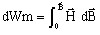which for a linear, homogeneous and isotropic problem can be also written in the form: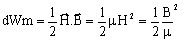The magnetic energy in a volume region is the integral: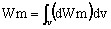## Magnetic force and torque

The magnetic force (torque) exerted on an indeformable part of the study domain can be computed by the virtual works method. The force (torque) in a given direction is obtained by deriving the system energy with respect to a virtual displacement of the part in this direction.

The magnetic force Fm in the direction is given by the following derivative: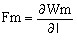where l is the elementary linear displacement in the direction.

The magnetic torque Γ m is given by the following derivative: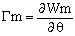where θ is the elementary angular displacement

In order to carry out this computation, the part must be surrounded by air. There can be massive regions or thin regions of Vacuum type.

## Density of surface force

The quantity DFSM is the density of the surface force (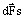) exerted between regions of different permeability. Thus, it is accessible only at the interface between the regions. The density of the surface force () is always normal to the surface.

The theoretical expression of the density of the surface force between two regions 1 and 2 is given by the relation: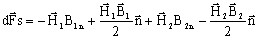In Flux, it is computed using the expression allowing the normality proper of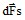, independently of the mesh and of the solution quality, i.e. by the relation: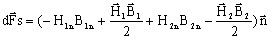If the magnetic material has a linear characteristic, we can define a surface force, or magnetic pressure Pm expressed by the integral: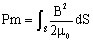The integral of DFSM on the group of external faces of the magnetic volume regions gives the global force on these regions. The precision obtained is theoretically lower than that obtained by the virtual works method.

## Laplace's force

Laplace's force is the force that is exerted on a current carrying conductor placed in a magnetic field.

The density of Laplace's force in a point is expressed by means of the current density J and the magnetic flux density B by the relation: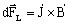Laplace's force in a volume region is the integral: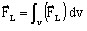The computation of Laplace's force exerted on a conductor is achievable when it is possible to get the value of J in all the points of this conductor.

• In the case of non-meshed type coils, the value of the current source in all the points of the coil are not directly provided by Flux. Thus, the computation of Laplace's force is not directly possible.
• In the case of meshed type coils (regions of coil conductor type), the value of the current source is provided in all the points of the coil (JS1), Therefore the computation is achievable.